# Maharashtra Board 12th Maths Solutions Chapter 3 Trigonometric Functions Ex 3.1

Balbharti 12th Maharashtra State Board Maths Solutions Book Pdf Chapter 3 Trigonometric Functions Ex 3.1 Questions and Answers.

## Maharashtra State Board 12th Maths Solutions Chapter 3 Trigonometric Functions Ex 3.1

Question 1.
Find the principal solutions of the following equations :
(i) cos θ= $$\frac{1}{2}$$
Solution:
We know that, cos$$\frac{\pi}{3}$$ = $$\frac{1}{2}$$ and cos (2π – θ) = cos θ
∴ cos$$\frac{\pi}{3}$$ = cos(2π – $$\frac{\pi}{3}$$) = cos$$\frac{5 \pi}{3}$$
∴ cos$$\frac{\pi}{3}$$ = cos$$\frac{5 \pi}{3}$$ = $$\frac{1}{2}$$, where
0 < $$\frac{\pi}{3}$$ < 2π and 0 < $$\frac{5 \pi}{3}$$ < 2π
∴ cos θ = $$\frac{1}{2}$$ gives cos θ = cos$$\frac{\pi}{3}$$ = cos$$\frac{5 \pi}{3}$$
∴ θ = $$\frac{\pi}{3}$$ and θ = $$\frac{5 \pi}{3}$$
Hence, the required principal solutions are
θ = $$\frac{\pi}{3}$$ and θ = $$\frac{5 \pi}{3}$$

(ii) sec θ = $$\frac{2}{\sqrt{3}}$$
Solution:

(iii) cot θ = $$\sqrt {3}$$
Solution:
The given equation is cot θ = $$\sqrt {3}$$ which is same as tan θ = $$\frac{1}{\sqrt{3}}$$.
We know that,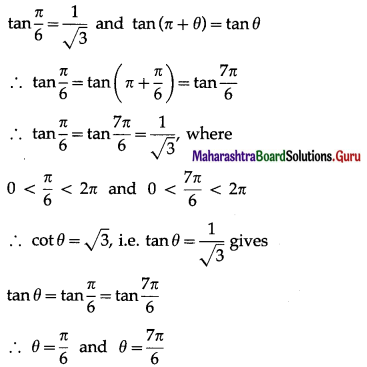Hence, the required principal solution are
θ = $$\frac{\pi}{6}$$ and θ = $$\frac{7 \pi}{6}$$.(iv) cot θ = 0.
Solution:

Question 2.
Find the principal solutions of the following equations:
(i) sinθ = $$-\frac{1}{2}$$
Solution:
We know that,
sin$$\frac{\pi}{6}$$ = $$\frac{1}{2}$$ and sin (π + θ) = -sinθ,
sin(2π – θ) = -sinθ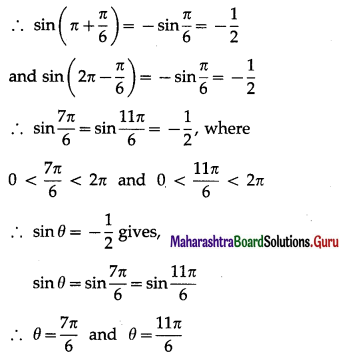Hence, the required principal solutions are
θ = $$\frac{7\pi}{6}$$ and θ = $$\frac{11 \pi}{6}$$.

(ii) tanθ = -1
Solution:
We know that,
tan$$\frac{\pi}{4}$$ = 1 and tan(π – θ) = -tanθ,
tan(2π – θ) = -tanθ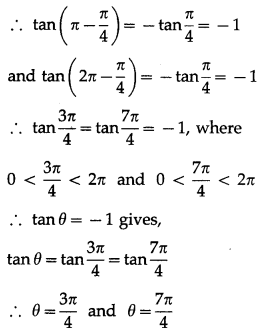Hence, the required principal solutions are
θ = $$\frac{3\pi}{4}$$ and θ = $$\frac{7 \pi}{4}$$.(iii) $$\sqrt {3}$$ cosecθ + 2 = 0.
Solution:

Question 3.
Find the general solutions of the following equations :
(i) sinθ = $$\frac{1}{2}$$
Solution:
(i) The general solution of sin θ = sin ∝ is
θ = nπ + (-1 )n∝, n ∈ Z
Now, sinθ = $$\frac{1}{2}$$ = sin$$\frac{\pi}{6}$$ …[∵ sin$$\frac{\pi}{6}$$ = $$\frac{1}{2}$$]
∴ the required general solution is
θ = nπ + (-1)n$$\frac{\pi}{6}$$, n ∈ Z.

(ii) cosθ = $$\frac{\sqrt{3}}{2}$$
Solution:
The general solution of cos θ = cos ∝ is
θ = 2nπ ± ∝, n ∈ Z
Now, cosθ = $$\frac{\sqrt{3}}{2}$$ = cos$$\frac{\pi}{6}$$ …[∵ cos$$\frac{\pi}{6}$$ = $$\frac{\sqrt{3}}{2}$$]
∴ the required general solution is
θ = 2nπ ± $$\frac{\pi}{6}$$, n ∈ Z.(iii) tanθ = $$\frac{1}{\sqrt{3}}$$
Solution:
The general solution of tan θ = tan ∝ is
θ = nπ + ∝, n ∈ Z
Now, tan θ = $$\frac{1}{\sqrt{3}}$$ = tan$$\frac{\pi}{6}$$ …[tan$$\frac{\pi}{6}$$ = $$\frac{1}{\sqrt{3}}$$]
∴ the required general solution is
θ = nπ + $$\frac{\pi}{6}$$ , n ∈ Z.

(iv) cotθ = 0.
Solution:
The general solution of tan θ = tan ∝ is
θ = nπ + ∝, n ∈ Z
Now, cot θ = 0 ∴ tan θ does not exist
∴ tanθ = tan$$\frac{\pi}{2}$$ [∵ tan$$\frac{\pi}{2}$$ does not exist]
∴ the required general solution is
θ = nπ + $$\frac{\pi}{2}$$, n ∈ Z.Question 4.
Find the general solutions of the following equations:
(i) secθ = $$\sqrt {2}$$
Solution:
The general solution of cos θ = cos ∝ is
θ = nπ ± ∝, n ∈ Z.
Now, secθ = $$\sqrt {2}$$ ∴ cosθ = $$\frac{1}{\sqrt{2}}$$
∴ cosθ = cos$$\frac{\pi}{4}$$ ….[cos$$\frac{\pi}{4}$$ = $$\frac{1}{\sqrt{2}}$$]
∴ the required general solution is
θ = 2nπ ± $$\frac{\pi}{4}$$, n ∈ Z.

(ii) cosecθ = –$$\sqrt {2}$$
Solution:
The general solution of sinθ = sin∝ is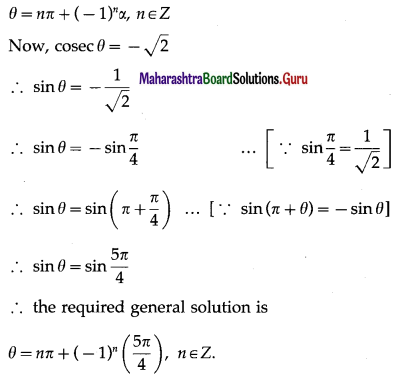(iii) tanθ = -1
Solution:
The general solution of tanθ = tan∝ is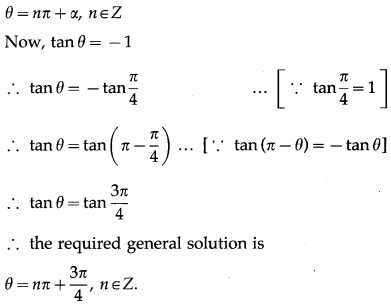Question 5.
Find the general solutions of the following equations :
(i) sin 2θ = $$\frac{1}{2}$$
Solution:
The general solution of sin θ = sin ∝ is
θ = nπ + (-1)n∝, n ∈ Z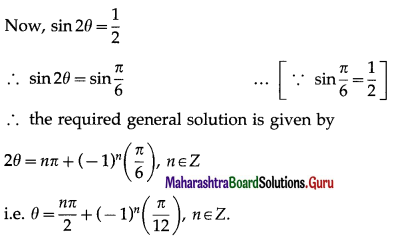(ii) tan $$\frac{2 \theta}{3}$$ = $$\sqrt {3}$$
Solution:
The general solution of tan θ = tan ∝ is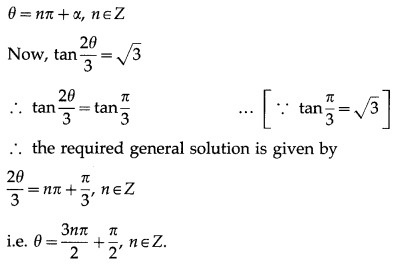(iii) cot 4θ = -1
Solution:
The general solution of tan θ = tan ∝ is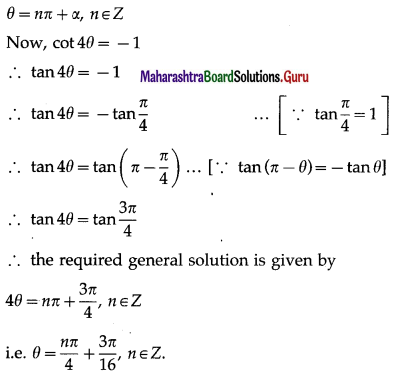Question 6.
Find the general solutions of the following equations :
(i) 4 cos2θ = 3
Solution:
The general solution of cos2θ = cos2 ∝ is
θ = nπ ± ∝, n ∈ Z
Now, 4 cos2θ = 3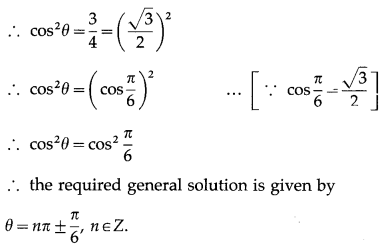(ii) 4 sin2θ = 1
Solution:
The general solution of sin2θ = sin2 ∝ is
θ = nπ ± ∝, n ∈ Z
Now, 4 sin2θ = 3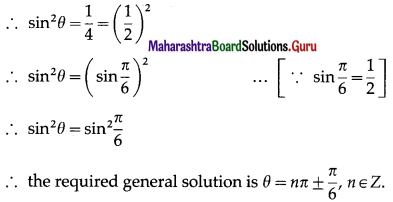(iii) cos 4θ = cos 2θ
Solution:
The general solution of cos θ = cos ∝ is
θ = 2nπ ± ∝, n ∈ Z
∴ the general solution of cos 4θ = cos 2θ is given by
4θ = 2nπ ± 2θ, n ∈ Z
Taking positive sign, we get
4θ = 2nπ + 2θ, n ∈ Z
∴ 2θ = 2nπ, n ∈ Z
∴ θ = nπ, n ∈ Z
Taking negative sign, we get
4θ = 2nπ – 2θ, n ∈ Z
∴ 6θ = 2nπ, n ∈ Z
∴ θ = $$\frac{n \pi}{3}$$, n ∈ Z
Hence, the required general solution is
θ = $$\frac{n \pi}{3}$$, n ∈ Z or ∴ θ = nπ, n ∈ Z.
Alternative Method:
cos 4θ = cos 2θ
∴ cos4θ – cos 20 = 0
∴ -2sin$$\left(\frac{4 \theta+2 \theta}{2}\right)$$∙sin$$\left(\frac{4 \theta-2 \theta}{2}\right)$$ = 0
∴ sin3θ∙sinθ = 0
∴ either sin3θ = 0 or sin θ = 0
The general solution of sin θ = 0 is
θ = nπ, n ∈ Z.
∴ the required general solution is given by
3θ = nπ, n ∈ Z or θ = nπ, n ∈ Z
i.e. θ = $$\frac{n \pi}{3}$$, n ∈ Z or θ = nπ, n ∈ Z.

Question 7.
Find the general solutions of the following equations :
(i) sinθ = tanθ
Solution:
sin θ = tan θ
∴ sin θ = $$\frac{\sin \theta}{\cos \theta}$$
∴ sin θ cos θ = sin θ
∴ sin θ cos θ – sinθ = 0
∴ sin θ (cos θ – 1) = θ
∴ either sinθ = 0 or cosθ – 1 = 0
∴ either sin θ = 0 or cos θ = 1
∴ either sinθ = 0 or cosθ = cosθ …[∵ cos0 = 1]
The general solution of sinθ = 0 is θ = nπ, n ∈ Z and cos θ = cos ∝ is θ = 2nπ ± ∝, where n ∈ Z.
∴ the required general solution is given by
θ = nπ, n ∈ Z or θ = 2nπ ± 0, n ∈ Z
∴ θ = nπ, n ∈ Z or θ = 2nπ, n ∈ Z.(ii) tan3θ = 3tanθ
Solution:
tan3θ = 3tanθ
∴ tan3θ – 3tanθ = 0
∴ tan θ (tan2θ – 3) = 0
∴ either tan θ = 0 or tan2θ – 3 = 0
∴ either tanθ = 0 or tan2θ = 3
∴ either tan θ = 0 or tan2θ = ($$\sqrt {3}$$ )3
∴ either tan θ = 0 or tan2θ = (tan$$\frac{\pi}{3}$$)3 …[tan$$\frac{\pi}{3}$$ = $$\sqrt {3}$$]
∴ either tanθ = 0 or tan2θ = tan2$$\frac{\pi}{3}$$
The general solution of
tanθ = 0 is θ = nπ, n ∈ Z and
tan2θ = tan2∝ is θ = nπ ± ∝, n ∈ Z.
∴ the required general solution is given by
θ = nπ, n ∈ Z or θ = nπ ± $$\frac{\pi}{3}$$, n ∈ Z.

(iii) cosθ + sinθ = 1.
Solution:
cosθ + sinθ = 1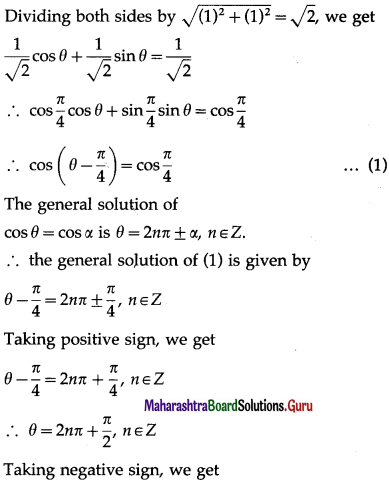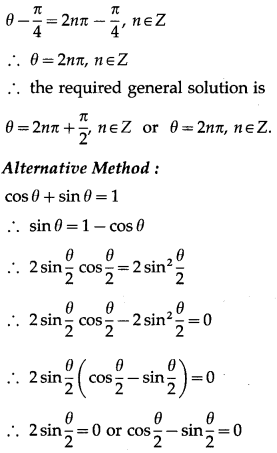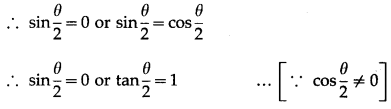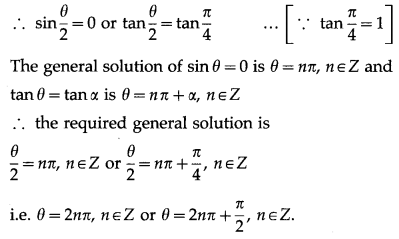Question 8.
Which of the following equations have solutions ?
(i) cos 2θ = -1
Solution:
cos 2θ = -1
Since -1 ≤ cos θ ≤ 1 for any θ,
cos 2θ = -1 has solution.(ii) cos2θ = -1
Solution:
cos2θ = -1
This is not possible because cos2θ ≥ 0 for any θ.
∴ cos2θ = -1 does not have any solution.

(iii) 2 sinθ = 3
Solution:
2 sin θ = 3 ∴ sin θ = $$\frac{3}{2}$$
This is not possible because -1 ≤ sin θ ≤ 1 for any θ.
∴ 2 sin θ = 3 does not have any solution.(iv) 3 tanθ = 5
Solution:
3tanθ = 5 ∴ tanθ = $$\frac{5}{3}$$
This is possible because tan θ is any real number.
∴ 3tanθ = 5 has solution.